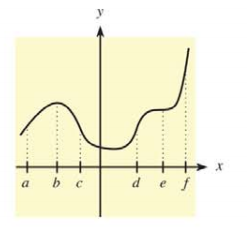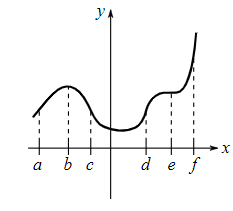Chapter 10.2, Problem 8E### Mathematical Applications for the ...

11th Edition
Ronald J. Harshbarger + 1 other
ISBN: 9781305108042

#### Solutions

Chapter
Section### Mathematical Applications for the ...

11th Edition
Ronald J. Harshbarger + 1 other
ISBN: 9781305108042
Textbook Problem

# In Problems 5-10, use the indicated x-values on the graph of y = f ( x ) to find the following.Find the x-coordinate of a horizontal point of inflection.

To determine

The x coordinates of horizontal points of inflection.Explanation

Given Information:

The provided graph is,

Explanation:

Consider the provided graph,

A point (x,f(x)) on the graph of function (x,f(x)) said to be point of inflection if the graph of function is concave up on one side of the point and concave down on the other side or the second derivative at point

### Still sussing out bartleby?

Check out a sample textbook solution.

See a sample solution

#### The Solution to Your Study Problems

Bartleby provides explanations to thousands of textbook problems written by our experts, many with advanced degrees!

Get Started